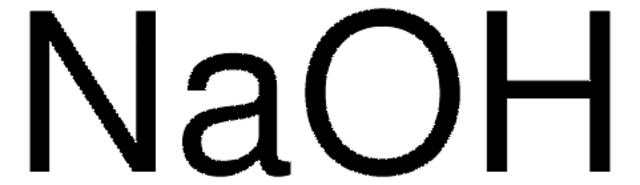# Sodium hydroxide solutionLinear Formula:
NaOH
CAS Number:
Molecular Weight:
40.00

## Product Comparison Guide

Use the product attributes below to configure the comparison table. (Select up to 3 total.)

Select Attribute
Select Attribute
Select Attribute
Sort by: Default
Product Number
Product Description
Pricing

### 1.37060

Sodium hydroxide solution, EMPROVE® EXPERT, suitable for cleaning in place, 0,5 mol/L

### 72068

Sodium hydroxide solution, BioUltra, for molecular biology, 10 M in H2O

### 415413

Sodium hydroxide solution, 50% in H2O

### 1.09137

Sodium hydroxide solution, c(NaOH) = 1 mol/l (1 N), Titripur®, reag. Ph. Eur., reag. USP

### S2770

Sodium hydroxide solution, 1.0 N, BioReagent, suitable for cell culture

### 1.09141

Sodium hydroxide solution, c(NaOH) = 0.1 mol/l (0.1 N), Titripur®, reag. Ph. Eur., reag. USP

### 72064

Sodium hydroxide solution, 49-51% in water, eluent for IC

### 1.09959

Sodium hydroxide solution, for 1000 ml, c(NaOH) = 0.1 mol/l (0.1 N) Titrisol®

### 1.09138

Sodium hydroxide solution, c(NaOH) = 0.5 mol/l (0.5 N), Titripur®

### S8263

Sodium hydroxide solution, 5.0 M

### 79724

Sodium hydroxide solution, 1 M, for HPCE

### 137031

Sodium hydroxide solution, 1 M (mol/L)

### 1.09136

Sodium hydroxide solution, c(NaOH) = 2 mol/l (2 N), Titripur®

### 1.09956

Sodium hydroxide solution, for 1000 ml, c(NaOH) = 1 mol/l (1 N) Titrisol®

### 1.09139

Sodium hydroxide solution, c(NaOH) = 0.25 mol/l (0.25 N), Titripur®

### 69413

Sodium hydroxide solution, suitable for HPLC, LiChropur, 49.0-51.0%

### 1.37041

Sodium hydroxide solution, 5 M in solution, EMPROVE® EXPERT

### 1.09140

Sodium hydroxide solution, c(NaOH) = 0.2 mol/l (0.2 N), Titripur®

### 1.09142

Sodium hydroxide solution, c(NaOH) = 0.02 mol/l (0.02 N), Titripur®

### 1.09957

Sodium hydroxide solution, for 1000 ml, c(NaOH) = 0.5 mol/l (0.5 N) Titrisol®

### 1.09961

Sodium hydroxide solution, for 1000 ml, c(NaOH) = 0.01 mol/l (0.01 N) Titrisol®

### 1.37058

Sodium hydroxide solution, 0,1 M, EMPROVE® EXPERT, suitable for cleaning in place

### 1.11584

Sodium hydroxide solution, c(NaOH) = 4 mol/l (4 N), Titripur®

### 150705

Sodium hydroxide solution, 0.1 M, Set incl. Absorber cartridge with soda lime, for 3S adapter technology

### 150706

Sodium hydroxide solution, 1 M, Set incl. Absorber cartridge with soda lime, for 3S adapter technology

### 1.09958

Sodium hydroxide solution, for 1000 ml, c(NaOH) = 0.25 mol/l (0.25 N) Titrisol®

### 58300C

Sodium hydroxide solution, 0.1 M

### 58310C

Sodium hydroxide solution, 0.5 M

### 58320C

Sodium hydroxide solution, 1 M

### 1.05595

Sodium hydroxide solution, c(NaOH) = 0.33 mol/l (1/3 N), Titripur®